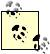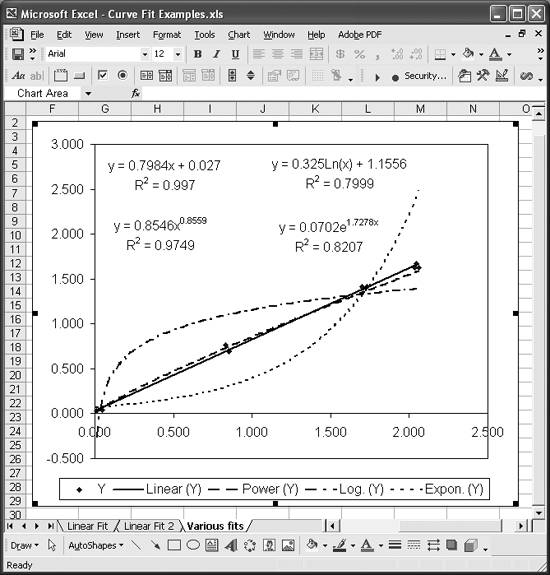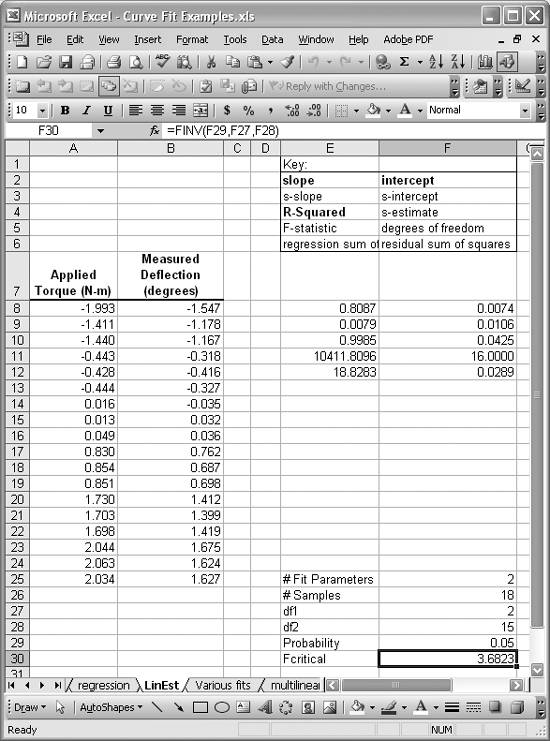# Assessing Goodness of Fit

Problem

You need to assess how well an equation fits the underlying data.

Solution

If you're simply fitting a curve through some data so you can conveniently interpolate the data, then choose whichever model best replicates your data. In this case you're not so concerned with smoothing the data or with statistical rigor. You can plot your data along with estimates using the fit curve and eyeball them to see how well they compare. You can also plot the residualsdifferences between your actual y-value and the estimated y-valueand examine them to assess how well your data is represented or to determine if the residuals exhibit some unexpected structure. You can compute percentage differences to gauge how well your data is replicated. Further, you should examine how the trendline behaves between data points used to generate the trendline to make sure there are no unrealistic oscillations between data points. This can happen when you try to fit a higher-order polynomial trendline.

If you're interested in modeling the data from a statistical standpoint, or are trying to gain insight into a physical process, then you can perform some standard statistical calculations to help assess your trendline. Moreover, you should consider what your data represents. If your data represents some physical process, then let physics be your guide and choose a model that best represents the physical relationship between the variables underlying the data, if it is indeed known. In the discussion to follow, I show you how to perform several standard calculations for assessing goodness of fit in Excel.

Discussion

As already mentioned, one way to see how well your equation fits the underlying data is to plot the fit equation over the data and observe how well it appears to capture the relationship between the variables considered. The correlation coefficient is a quantitative way to assess how well your equation models the relationship between the underlying variables.

Any standard text on statistical analysis that covers regression and correlation theory can provide details on the derivation of the correlation coefficient. In a nutshell, the correlation coefficient is a ratio of variations between the variables estimated using the fit equation and the actual variables. The basic formula for correlation coefficient is:The term in the denominator under the radical is called the total variation of Y. It's a measure of the deviations of Y from the mean value of Y in the dataset. Total variation is composed of two components: the explained variation and the unexplained variation. Explained variation is a measure of the deviations of the estimated Y values (those predicted from the fit curve) from the mean value of Y in the dataset. Unexplained variation is a measure of the deviations of the estimated Y value from the actual or measured Y value. These variations are assumed random and it's these variations you aim to minimize when fitting a curve to a set of data.The formula for the correlation coefficient can be and often is written in different forms. For example, in some cases it's written in terms of standard deviations.

As you can see from the formula shown a moment ago, the correlation coefficient is the ratio of the explained variation to the total variation. The greater the explained variation, the smaller the unexplained variation, and therefore the closer the correlation coefficient approaches a value of ±1. The R-squared value is just the square of the correlation coefficient. Therefore, the closer R-squared is to a value of 1, the smaller the unexplained variation and the better the fit. Excel's curve fit and trendline functions compute the R-squared value, which is also called the coefficient of determination . I've mentioned this R-squared value several times in other recipes already. The closer the R-squared value is to 1, the better the equation fits the underlying data.You can display the R-squared value for a chart trendline by checking "Display R-squared value on chart" under the Options tab in the Add Trendline dialog box. See Figure 8-2 in Recipe 8.1.

For illustration purposes, I prepared a dataset that exhibits a linear relationship and had Excel compute various trendlines, including one linear and three nonlinear trendlines. The results are shown in Figure 8-13.

The equations, along with the corresponding R-squared values, are also shown on the chart. The linear trendline best fits the data, as expected, with an R-squared value of 0.997. The next best fit is from the power fit, with an R-squared value of 0.9749, which is still not a bad fit. The other two trendlinesthe log and exponential

Figure 8-13. Various trendlinestrendlinesexhibit poor fits, with R-squared values of 0.7999 and 0.8207, respectively. You can also visually see that the log and exponential fits poorly represent the data under consideration.

Examining these results brings up a point of concern. Say you initially fit a power curve to the data shown here. You might reasonably assume the power curve is the best-fit curve, given the resulting R-squared value of 0.9749. However, we happen to know a little something about the physical phenomenon this data represents and know that this is a linear problem. A linear fit indeed shows a better fit, with an R-squared value of 0.997. These results reinforce the importance of the need to use your judgment and understanding of the problem being analyzed when choosing an appropriate model.

As a further check on how well a model equation fits the data, you can plot the residuals. (See Recipe 8.6 for an example showing how to compute residuals.) These represent the unexplained variation between the estimated y-values and the given y-values. Unexplained variations are supposed to be random, and the residuals, when plotted, should confirm this behavior. If you plot the residuals and see some structure to the residual plot that does not appear random, you should take a closer look at your assumed model.

As an illustrative example, consider the data shown in Figure 8-12 along with the linear and exponential trendlines. The residuals between the linear fit and the exponential fit are plotted in Figure 8-14.

Figure 8-14. Residual plotThe residual curve from the linear fit does seem to exhibit a random pattern. On the other hand, the residual curve from the exponential fit exhibits a very distinct pattern, resembling an exponential curve that's sort of been shifted down a bit. Such a structure in the residual plot indicates a fundamental misrepresentation of the underlying data. This is an exaggerated example, as we already knew the exponential curve was a poor fit. However, the point is that examining the residual plot can give you some insight into the goodness of the fit.

Another check on how well a model represents a set of data is known as the F-test . This test comes from a technique known as analysis of variance (ANOVA) developed by R. A. Fisher. The formal details of this test can be found in standard statistical texts that cover ANOVA.

Excel makes it easy to perform this F-test because its regression functions, such as LINEST, return the statistics required for this test, and Excel also contains other required probability-related functions. The F-test basically involves computing a statistic known as the F-statistic and comparing that value to a critical value obtained from the standard F-distribution using Excel's built-in functions. If the computed F-statistic is greater than the critical statistic, then you can conclude the fit is a good one or, more formally, statistically significant.

Figure 8-15 shows an example linear curve fit using Excel's LINEST function (see Recipe 8.3). The results of using LINEST on the given dataset are contained in the cell range E8:F12. I've included a key explaining what each returned value represents. The value in cell E11 is the F-statistic computed by LINEST.

Cells F25 to F30 contain calculations I added to compute the critical F-value for comparison. Cell F25 contains the number of parameters in the regression equation; 2 in this case, since it's linear. Cell F26 contains the number of samples. I used =COUNT(A8:A25) to determine the number of samples (of course, for this small dataset they can be easily counted manually). Cell F27 contains the numerator degrees of freedom required for computing F-critical; this value is equal to the number of parameters in the regression equation. Cell F28 contains the denominator degrees of freedom, which is equal to n - (k + 1), where n is the number of samples and k is the number of regression parameters. Cell F29 contains the probability of significance for which we're interested in computing F-critical. F-critical is computed using the formula =FINV(F29,F27,F28). In this case, we get an F-critical value of 3.6823, which is far lower than the F-statistic computed for the curve fit. This indicates that curve fit is significant and useful in representing the underlying data.FINV is a built-in formula that returns the inverse of the F-distribution. See Excel's help topic "FINV" and Recipe 5.6 for more information.

Assessing goodness of fit is certainly not limited to the techniques I've discussed here. I'm sure a professional statistician could easily explain a half-dozen more statistical tests that could be used to validate a particular curve fit.

Figure 8-15. F-test exampleExcel Scientific and Engineering Cookbook (Cookbooks (OReilly))
ISBN: 0596008791
EAN: 2147483647
Year: N/A
Pages: 206
Authors: David M Bourg